## Connection Coefficient

A quantity also known as a Christoffel Symbol of the Second Kind. Connection Coefficients are defined by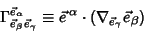(1)

(long form) or(2)

(abbreviated form), and satisfy(3)

(long form) and(4)

(abbreviated form).

Connection Coefficients are not Tensors, but have Tensor-like Contravariant and Covariant indices. A fully Covariant connection Coefficient is given by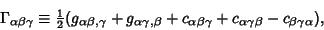(5)

where thes are the Metric Tensors, thes are Commutation Coefficients, and the commas indicate the Comma Derivative. In an Orthonormal Basis,and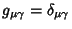, so(6)

and(7)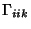(8)(9)(10)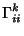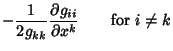(11)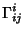(12)

For Tensors of Rank 3, the connection Coefficients may be concisely summarized in Matrix form:(13)

Connection Coefficients arise in the computation of Geodesics. The Geodesic Equation of free motion is(14)

or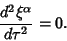(15)

Expanding,(16)(17)

But(18)

so(19)

where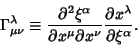(20)

See also Cartan Torsion Coefficient, Christoffel Symbol of the First Kind, Christoffel Symbol of the Second Kind, Comma Derivative, Commutation Coefficient, Curvilinear Coordinates, Semicolon Derivative, Tensor